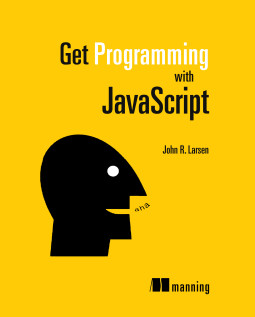## JavaScript Utilities: the between function

The between utility function uses Math.random to generate random whole numbers between two specified limits.

### Listing 1

```function between (lower, upper) { var scale = upper - lower + 1; return Math.floor(lower + Math.random() * scale); } // generate a whole number between 1 and 10, inclusive var num1 = between(1, 10); // generate a whole number between 5 and 10, inclusive var num2 = between(5, 10);```

num1 is assigned a whole number between 1 and 10, with both 1 and 10 as possible values.

num2 is assigned a whole number between 5 and 10. There are 6 possible values: 5, 6, 7, 8, 9, and 10.

### Generating a random number

Use the JavaScript Math.random method to generate a random number greater than or equal to 0 but less than 1. Listing 2 shows three possible values returned by Math.random.

### Listing 2

```Math.random(); // 0.7439713935034316 Math.random(); // 0.9362357408054629 Math.random(); // 0.8929897816872104```

### Generating a random number in a given interval

Multiply your number to scale it to the size you need. Listing 3 shows three random numbers greater than or equal to 0 but less than 10.

### Listing 3

```Math.random() * 10; // 3.3403217410674415 Math.random() * 10; // 1.8471253975128377 Math.random() * 10; // 8.280692620890159```

Add a starting value to your number to start the interval at a number other than zero. Listing 4 shows three random numbers greater than or equal to 10 but less than 20.

### Listing 4

```10 + Math.random() * 10; // 16.13400536878539 10 + Math.random() * 10; // 14.921878407083668 10 + Math.random() * 10; // 14.800218021993242```

### Generating a random whole number in a given interval

Use Math.floor to round your number down to the previous whole number. Listing 5 shows three numbers rounded down.

### Listing 5

```Math.floor(3.3403217410674415); // 3 Math.floor(1.8471253975128377); // 1 Math.floor(-8.280692620890159); // -9```

Listing 6 shows three random whole numbers greater than or equal to 10 but less than 20.

### Listing 6

```Math.floor(10 + Math.random() * 10); // 16 Math.floor(10 + Math.random() * 10); // 14 Math.floor(10 + Math.random() * 10); // 14```

### The between function

The between function generates random whole numbers between two specified limits. Both limits are inclusive - they are both possible values.

### Listing 7

```function between (lower, upper) { var scale = upper - lower + 1; return Math.floor(lower + Math.random() * scale); }```

The function first calculates the scale the raw random number should be multiplied by. The +1 is needed to make the upper limit inclusive. For example, if lower is 5 and upper is 10: scale = 10 - 5 + 1 = 6. There are 6 possible values: 5, 6, 7, 8, 9, and 10.

### Note

• You could check that lower and upper are in the right order and swap them if not.
• You could make sure lower and upper are whole numbers.
• Don't use Math.round. The between function would then return the lower and upper values half as often as other values in the interval.

## Get Programming with JavaScript

Keep learning! Get Programming with JavaScript is my new book, published by Manning and available in print and as an ebook.